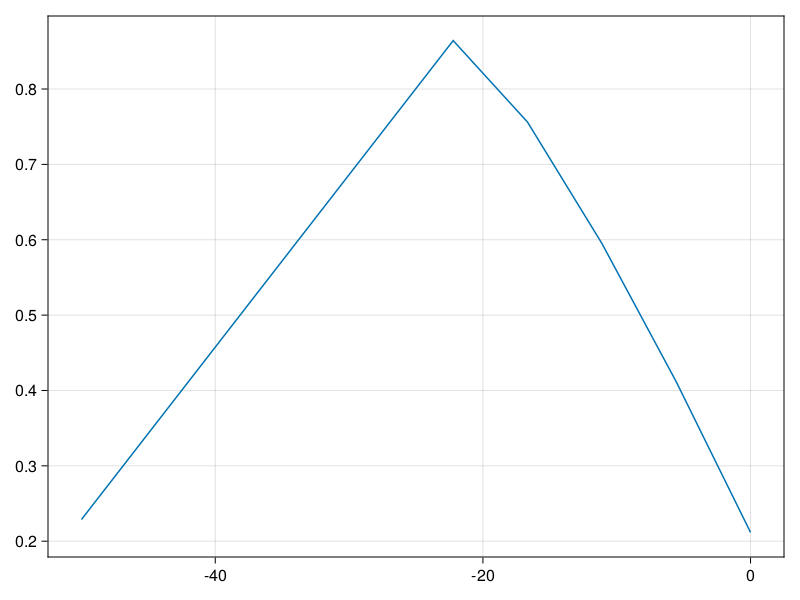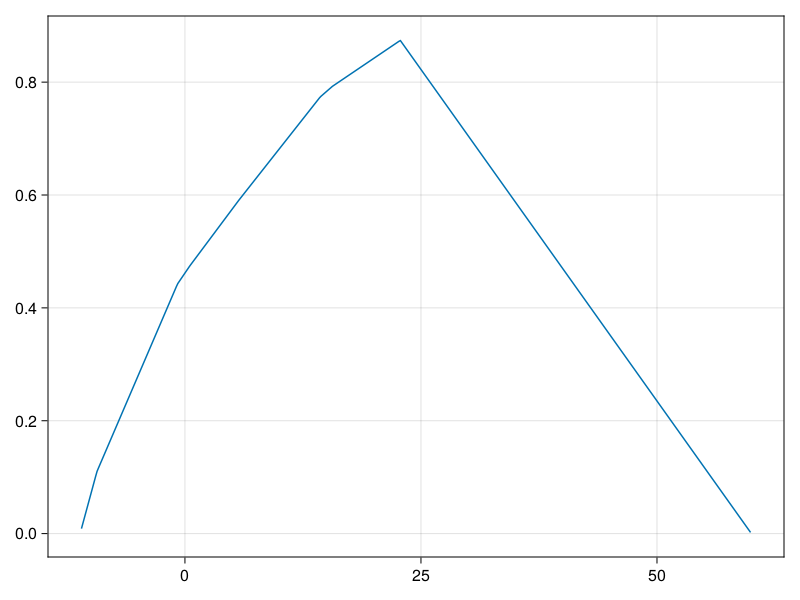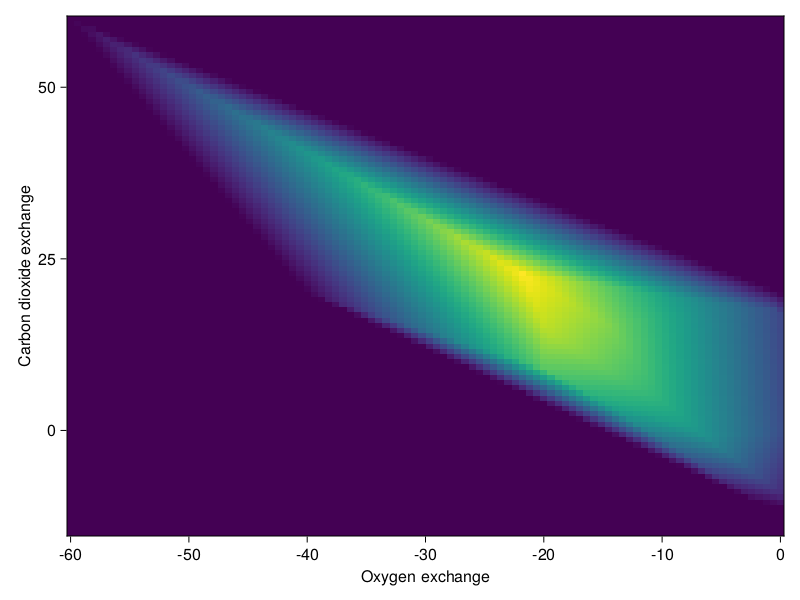# Production envelopes

Production envelopes show what a model is capable of doing on a wide range of parameters. Usually, you choose a regular grid of a small dimension in the parameter space, and get an information about how well the model runs at each point.

!isfile("e_coli_core.xml") &&

using COBREXA, GLPK

model = load_model("e_coli_core.xml")
Metabolic model of type SBMLModel
sparse([8, 10, 21, 43, 50, 51, 8, 9, 6, 12  …  33, 66, 68, 72, 23, 26, 33, 72, 22, 33], [1, 1, 1, 1, 1, 1, 2, 2, 3, 3  …  93, 93, 93, 93, 94, 94, 94, 94, 95, 95], [-1.0, 1.0, -1.0, 1.0, -1.0, 1.0, 1.0, -1.0, -1.0, 1.0  …  1.0, -1.0, 1.0, -1.0, -1.0, 1.0, 1.0, -1.0, -1.0, 1.0], 72, 95)
Number of reactions: 95
Number of metabolites: 72


The envelope analysis "structure" is similar to what you can obtain using screen; but COBREXA provides a special functions that run the process in a very optimized manner. For envelopes, there is envelope_lattice that generates the rectangular lattice of points for a given model and reactions, and objective_envelope that computes the output (usually as the objective value) of the model at the lattice points. You do not need to call envelope_lattice directly because it is taken as a "default" way to create the lattice by objective_envelope.

In short, we can compute the envelope of a single reaction in the E. coli model as follows:

envelope = objective_envelope(
model,
["R_EX_o2_e"],
GLPK.Optimizer,
lattice_args = (ranges = [(-50, 0)],),
)
(lattice = [[-50.0, -44.44444444444444, -38.888888888888886, -33.333333333333336, -27.77777777777778, -22.22222222222222, -16.66666666666667, -11.111111111111114, -5.555555555555557, 0.0]], values = [0.22877222522165902, 0.3558679059003752, 0.48296358657909405, 0.6100592672578073, 0.7371549479365156, 0.864250628615226, 0.7560960306233718, 0.5951579819836802, 0.4116468402637565, 0.21166294973531483])

(The named tuple given in lattice_args argument is passed to the internal call of envelope_lattice, giving you an easy way to customize its behavior.)

The result has 2 fields which can be used to easily plot the envelope. We also need to "fix" the missing values (represented as nothing) where the model failed to solve – we will simply omit them here).

using CairoMakie

valid = .!(isnothing.(envelope.values))
lines(envelope.lattice[valid], float.(envelope.values[valid]))Additional resolution can be obtained either by supplying your own larger lattice, or simply forwarding the samples argument to the internal call of envelope_lattice:

envelope = objective_envelope(
model,
["R_EX_co2_e"],
GLPK.Optimizer,
lattice_args = (samples = 1000, ranges = [(-50, 100)]),
)

valid = .!(isnothing.(envelope.values))
lines(envelope.lattice[valid], float.(envelope.values[valid]))## Multi-dimensional envelopes

The lattice functions generalize well to more dimensions; you can easily explore the production of the model depending on the relative fluxes of 2 reactions:

envelope = objective_envelope(
model,
["R_EX_o2_e", "R_EX_co2_e"],
GLPK.Optimizer,
lattice_args = (samples = 100, ranges = [(-60, 0), (-15, 60)]),
)

heatmap(
envelope.lattice,
envelope.lattice,
[isnothing(x) ? 0 : x for x in envelope.values],
axis = (; xlabel = "Oxygen exchange", ylabel = "Carbon dioxide exchange"),
)